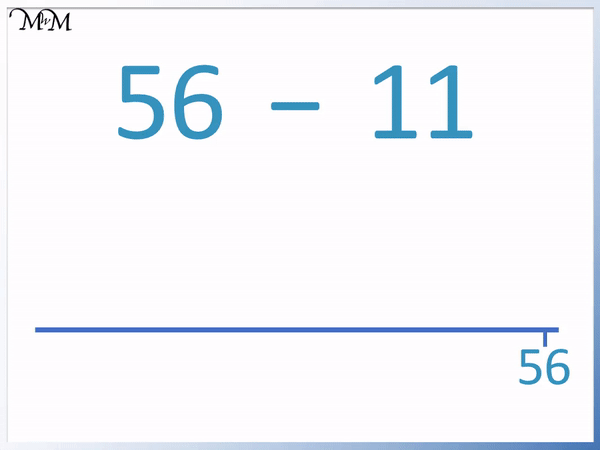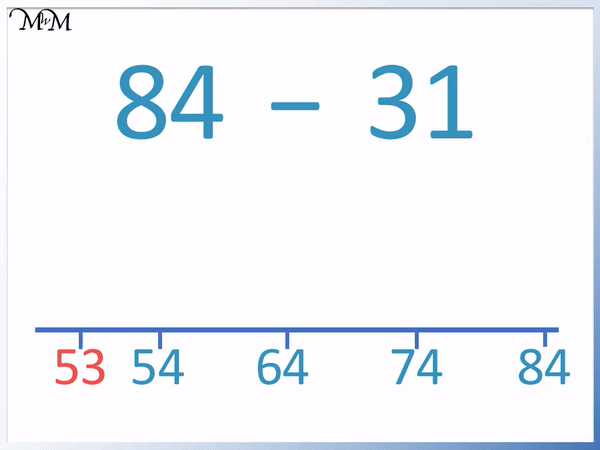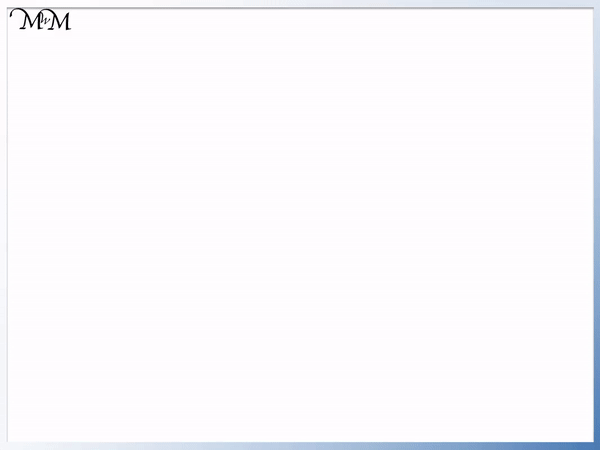# Subtraction on an Empty Number Line

Subtraction on an Empty Number Line• To subtract using the empty number line method, first draw a blank number line.
• Mark the largest number on the right of the number line.
• 84 is the number we are subtracting from so we write this first.
• The number we are subtracting is broken down into its tens and ones.
• 31 has 3 tens and 1 one.
• We subtract the tens first, in this case we make 3 subtractions in jumps of 10.
• 10 is easy to subtract because the ones digit remains the same but we decrease the tens digit by 1.
• 84 – 10 = 74, 74 – 10 = 64 and then 64 – 10 = 54.
• We now show the subtraction of the 1 unit with a smaller jump.
• 54 – 1 = 53
• This jump strategy is used to break down an subtraction and show the process visually.

Mark the number being subtracted from on the right of a number line.

Make jumps in tens and then ones to break down the subtraction.• 97 is the number we are subtracting from so we mark it on the right of the number line.
• 64 contains 6 tens and 4 ones.
• We can subtract 6 tens directly by decreasing the tens digit by 6.
• 9 – 6 = 3 and so, 97 – 60 = 37.
• We show the subtraction with a jump to the left on our number line from 97 to 37.
• We now subtract the 4 ones.
• 7 – 4 = 3 and so, 37 – 4 = 33.
• We draw a smaller jump from 37 to 33 on the number line.# Subtraction on an Empty Number Line

## What is the Empty Number Line Strategy?

The empty number line strategy is a method which shows an addition or subtraction visually, using jumps in tens or ones.

The empty number line strategy is also known as the jump strategy, open number line or blank number line strategy. The empty number line strategy is useful because it helps people to visualise the size of each number in the different stages in their calculation.

In this lesson we will use the empty number line strategy for subtraction.

Here is an example of a 2-digit subtraction using a number line.

We have 56 – 11.

The number line is important in breaking down the subtraction and showing the relative size of each number.

We need to subtract 11 so we break it down into 10 and 1.We subtract the ten and then the one separately.

Write the values after each subtraction on the number line. This helps to keep a running record of the calculation. The numbers written help us to visualise the size of each number and improves our ability to estimate and check our work.

Here is another example of using the blank number line method.

We have 84 – 31.

Subtraction on a number line can be performed in several ways. There is no one correct way to break the subtraction down.

When first teaching subtraction on a number line, it is useful to break the subtraction down into tens and ones.

We can break this subtraction of 31 into 3 tens and 1 one.

Here we subtract 10 three times.We then subtract the one.

When teaching subtraction on a number line, it is easier to subtract smaller numbers. Subtracting 10 is easy as it is just the tens digit that decreases by 1. Subtracting 1 is also easy.

Whilst the subtractions are easy, 4 jumps were needed to subtract 31.

As we progress with the method, we can use fewer and fewer jumps.

A common strategy is to break the subtraction down into multiples of 10 and 1.

31 is made of 3 tens and 1 one.

We could have done the subtraction by taking away 30 and then taking away 1.We can see that this is a different way of using the empty number line strategy. Fewer jumps were used but the final answer is the same.

## Subtraction using a Number Line

To subtract using a number line, use the following steps:

1. Draw a blank number line with no numbers written.
2. Write the number you are subtracting from on the right hand side of this line.
3. Read the digit in the tens column of the number being subtracted.
4. Subtract this digit from the tens digit of your original number.
5. Draw an arrow jump left going from the original number to this answer.
6. Read the digit in the ones column of the number being subtracted.
7. Subtract this digit from the ones digit of the previous answer.
8. Draw an arrow jump left from the previous answer to this new answer.

Here is an example of subtracting a 2-digit number using an open number line.

We have 97 – 64.

The first step is to draw the blank number line.

The second step is to mark the number we are subtracting from on the right hand side. We mark 97.The third step is to read the tens digit of 64, which is 6. We will subtract 6 tens.

The fourth step is to subtract the 6 from the tens digit of 97.

9 – 6 = 3 and so, 97 – 60 = 37.

The fifth step is to draw this subtraction on the number line using a jump.

The sixth step is to read the ones digit of 64, which is 4.

The seventh step is to subtract this digit from the ones digit of 37.

7 – 4 = 3 and so, 37 – 4 = 33.

Here is another example of working out a 2-digit subtraction on a number line using the jump strategy.

We have 82 – 54.

We can show the subtraction of the tens digit with one jump.

8 – 5 is 3 and so, 82 – 50 = 32.In this example we can see that if we went to subtract the 4 in one go, it can be a little difficult for some students.

This is because 4 is larger than 2 and subtracting 4 from 32 involves crossing the tens barrier at 30.

It may be easier to subtract the 4 from 32 in 4 separate jumps of 1.

32 – 4 = 28 and so, 82 – 54 = 28.Now try our lesson on Comparing 2-Digit Numbers with Greater Than and Less Than where we learn how to compare two numbers.error: Content is protected !!